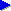Search this siteJava for beginners (topic index)Variablesfor (loops)ifelselogical operatorsarraysobjects# Arrays in Java

In our introduction to variables, we used the analogy of pigeon holes, and said that in general, a single variable took up a "pigeon hole" of memory, and that that pigeon hole had a particular label.

Sometimes, when we want to work with lists or groups of things, this notion of a single labelled pigeon hole per variable isn't very useful. For example, continuing with the idea of a card game that we've mentioned a couple of times, supposing we need to track the state of a given number of players. If we know that we have exactly two players, then to keep track of the number of cards that each player has in their hand, we could possibly declare two variables, numberOfCards1 and numberOfCards2. But the program is going to quickly get a bit awkward. Imagine that we need to find out the number of cards held by the current player. Well, we've seen that we can introduce conditions with the if statement, so we could just about write the following:

```int noCardsCurrentPlayer;
if (currentPlayer == 1) {
noCardsCurrentPlayer = noCardsPlayer1;
} else if (currentPlayer == 2) {
noCardsCurrentPlayer = noCardsPlayer2;
}
```

If we really had no other option, we could just about write things this way. But the program would soon get pretty ugly. Firstly, for every time we have to select a value depending on the player number, we'd need and if statement like this. And secondly, it's going to be difficult to make our program flexible— that is, essentially work with any number of players. We pretty much have to decide in advance how many players there are, and thus how many variables to declare, how many else if lines to write etc.

What we'd really like to do is say "reserve a row of x pigeon holes", where x is the number of players, and which we decide at the time of running the program rather than at the time of writing it. Then, when we want to know the number of cards in a particular player's hand, that is the value of the "xth pigeon hole" in the row we reserved. Well, that's effectively what an array is.

Let's assume that we have a variable, noPlayers, which holds the number of players in the current game. Now, to set aside a row of pigeon holes in memory to store the number of cards that each player has, we can write:

```int[] cardsPerPlayer = new int[noPlayers];
```

Note the addition of the square brackets [], which effectively mean "array of". At the beginning of the declaration, int[] means "array of int"— it's the type of variable that we're declaring, as before. Then in the second case, we inside the square brackets the number of ints that we want in the array: in this case, one for each player. Notice also that we've inserted the word new. We'll look in more detail at the keyword new, but it is essentially used to reserve some space in memory for something that isn't just a single, "simple" variable such as a single int.

So now, to refer to a particular "pigeon hole number" in the array (in programming, we'd often talk about a particular index or position in an array), we put the number inside square brackets, such as the following to refer to the first player or position in the array:

```cardsPerPlayer
```

or the following to refer to the number of cards of the current player:

```cardsPerPlayer[currentPlayer]
```

Notice that in fact, the positions in an array are numbered starting at zero, not 1. So in fact, if our player numbers started at 1, we'd end up writing:

```cardsPerPlayer[currentPlayer - 1]
```

Using a loop, the following would print out the number of cards held by each player in turn:

```for (int i = 1; i <= noPlayers; i++) {
int n = cardsPerPlayer[i - 1];
System.out.println("Player " + i + " has " + n + " cards");
}
```

Notice how with this version using an array and a loop, the actual program doesn't depend on the number of players, unlike the version with the separate variables and if statements above, where we'd need to add new lines of code for each new player we added. Similarly, we can set the number of cards held by each player as follows (here, we give each player 5 cards):

```for (int i = 0; i < noPlayers; i++) {
cardsPerPlayer[i] = 5;
}
```

Notice how in the previous example, the value of i went from 1 to the number of players inclusive, and on each pass through the loop, we subtracted one to convert this number into the array position. In this last example, we actually start counting at zero, and continue until one less than the number of players— in other words, this time, i is directly the array index and we don't need to subtract one. Why the two versions? Well, just because in the first case, we needed to display the player number as well as use it as an index. And to human beings, player numbers start at one, not zero! Of course, we could still have counted from zero, and added one to get the display number each time— in this case, as in many cases in programming, we have a choice, and neither option is necessarily preferable to the other.

In the second case, we're using i purely as an array index, so we may as well start counting at zero— i.e. "speak the computer's language" rather than the user's, since the human user will never see i in this case.

## Next: objects

We're going to come back to more examples of using arrays in a moment. But first, we'll take an excursion with our first look at objects, which will become a key concept in Java programming.